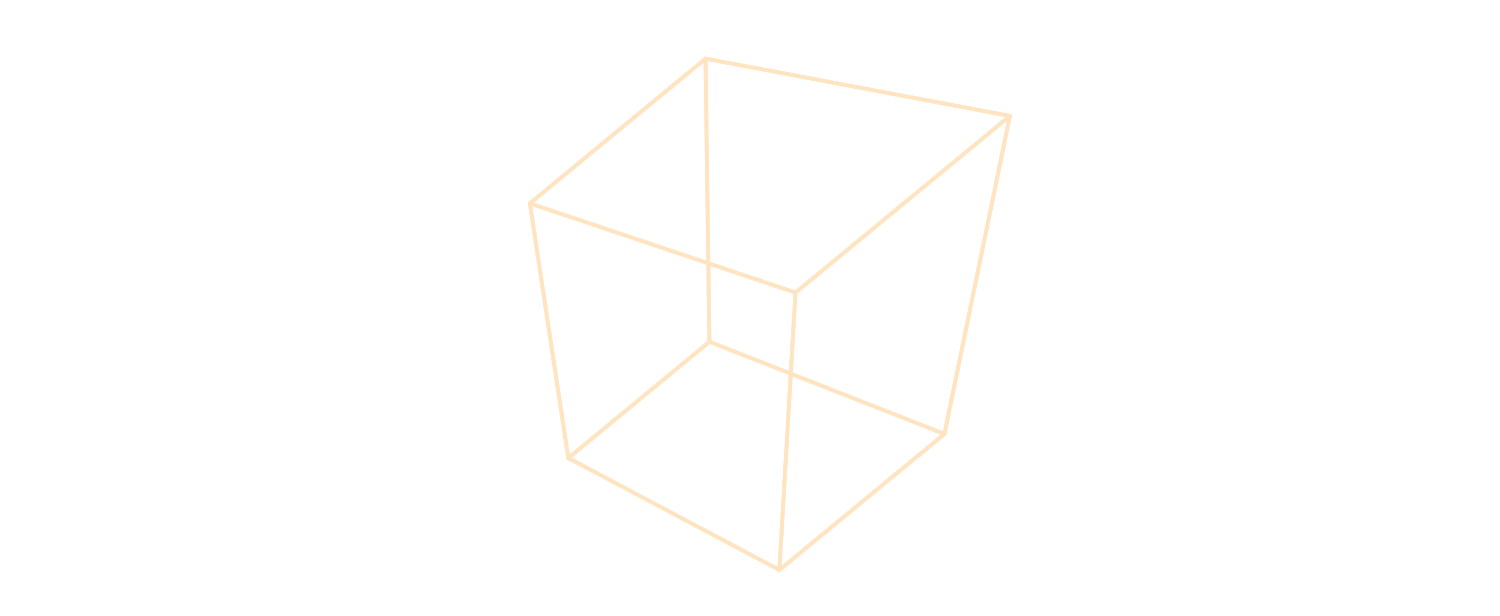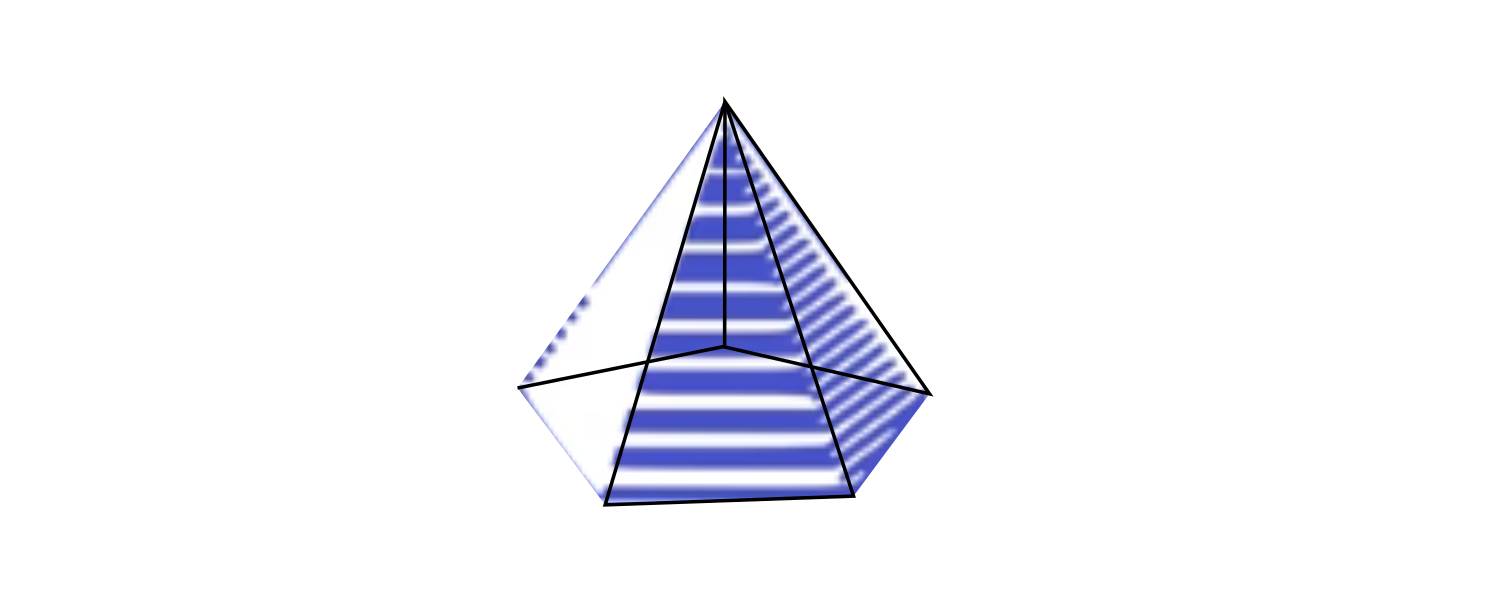# Question: What do V, E, and F represent here?

• What does V, E, and F represent here? I'm a bit confused because I'm pretty sure F is the middle pentagon, but I don't know about the rest.

• Lol my strategy doing this question:

pentagon in middle = 5 faces
pyramid with 3 faces per edge of pentagon = 15 faces
side triangles with 2 faces each = 10 faces
assuming there is a whole back layer to the shape = x2
(5+15+10) x 2 = 30 x 2 = 60

• @The-Blade-Dancer Thank you for this post; it's probably a good idea for us to annotate the video with the definitions of these variables, $$V, E, \text{ and } F,$$ and this is something we will put down to do in the future!I think you figured this out already!At any rate, I'll just give a quick answer.

For any 3-D shape made up of flat regular polygons (called a polyhedron), it's convenient to count its number of faces, vertices, and edges.

$$V$$ is the number of vertices in the polyhedron.
$$E$$ is the number of edges in the polyhedron.
$$F$$ is the number of faces in the polyhedron.For a cube, $$V = 8, E = 12,$$ and $$F = 6.$$

An edge is usually shared by two faces; a vertex can be shared by multiple faces.

If you count the number of faces and multiply by the sides per face, you will be counting each edge of the polyhedron twice.

If you count the number of faces and multiply by the number of corners per face, you will be counting each vertex of the polyhedron multiple times.

• @The-Blade-DancerThat's a really good way!I like it!Another slight variation on this method is to say that the 3-D figure is composed of the same repeated building-block, the pyramid with five triangular slant faces.On our side of the shape, there is a big 5-pointed-star looking at us, and it's composed of $$6$$ of these building-block pyramids, so the 5-pointed-star contains $$30$$ faces. The side facing opposite from us contains the same 5-pointed-star, simply rotated, so it provides another $$30$$ faces.

The total is $$30 + 30 = \boxed{60 \text{ faces}}.$$